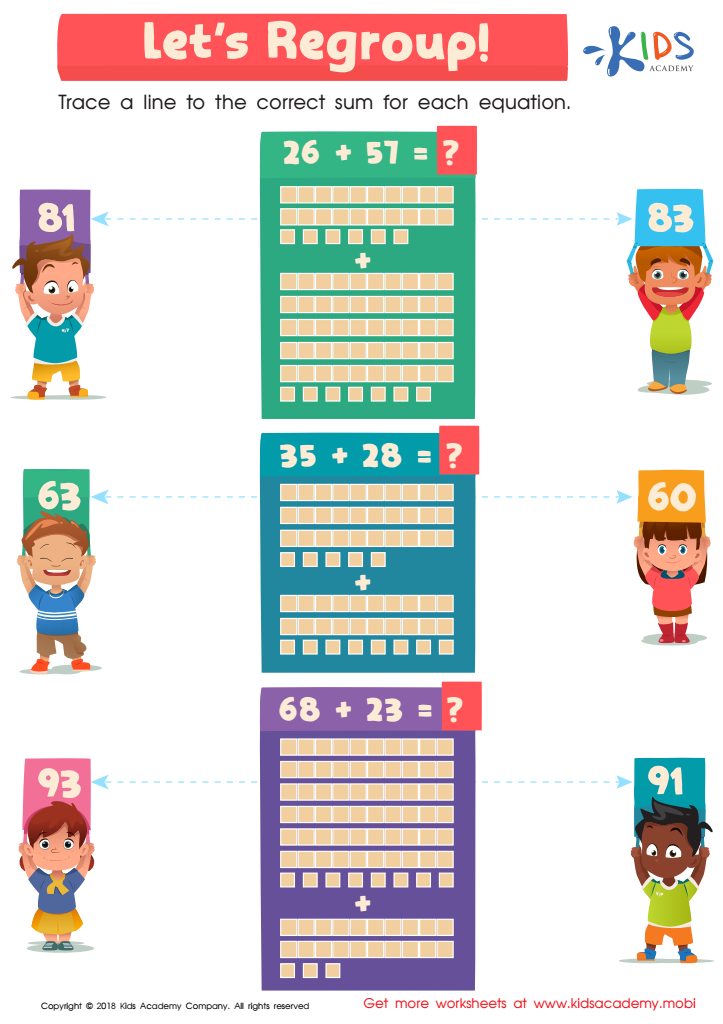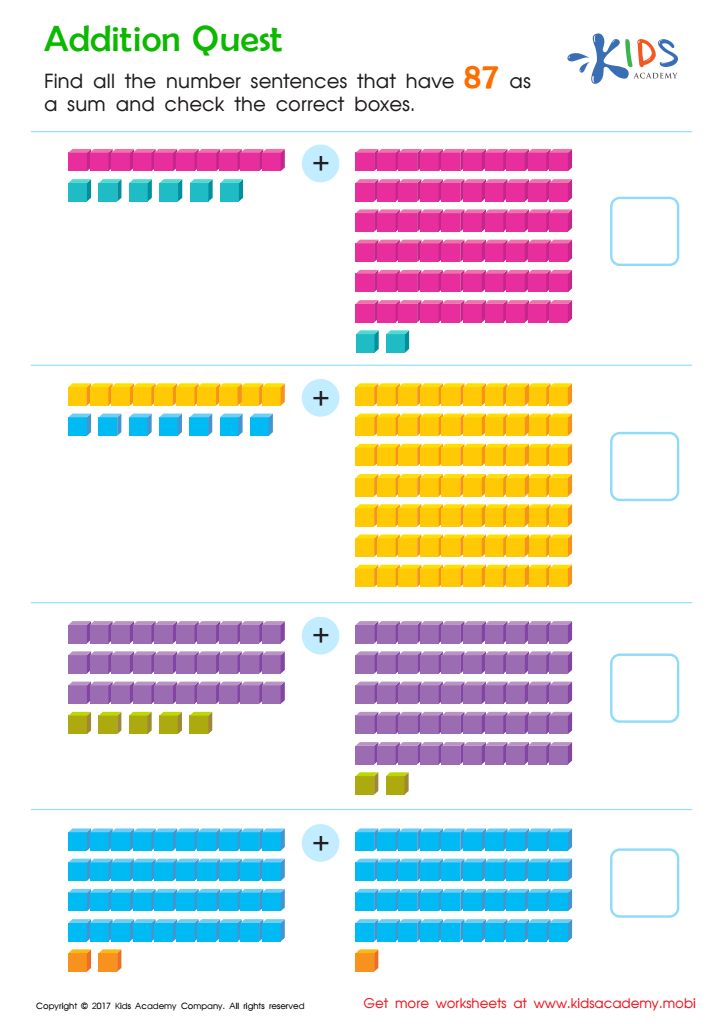# Extra Challenge Online Place Value Worksheets for Grade 1

6 filtered results

Clear all filters
Favorites
Interactive

6 filtered results

Difficulty Level

Standards
Favorites
Interactive
• Interactive
• Place Value
• Extra ChallengeLet's Regroup Worksheet
Counting is fun! Help teach your child with fun, colorful worksheets like this one. For simple sum-adding or counting high numbers, this printout helps them master the problems. Three equations with numbers are at the top. Guide your child to find the correct answer, then draw a line to the sum for each equation.
Let's Regroup Worksheet
WorksheetAddition and Subtraction Assessment 1 Worksheet
Kids often find math difficult, even adults! But it's important they learn the basics, like adding and subtracting. Use this worksheet to help them practice. Check the box next to the correct answer for each equation. It's a great way to give them a jump start and help them succeed in math.
Addition and Subtraction Assessment 1 Worksheet
Worksheet